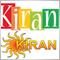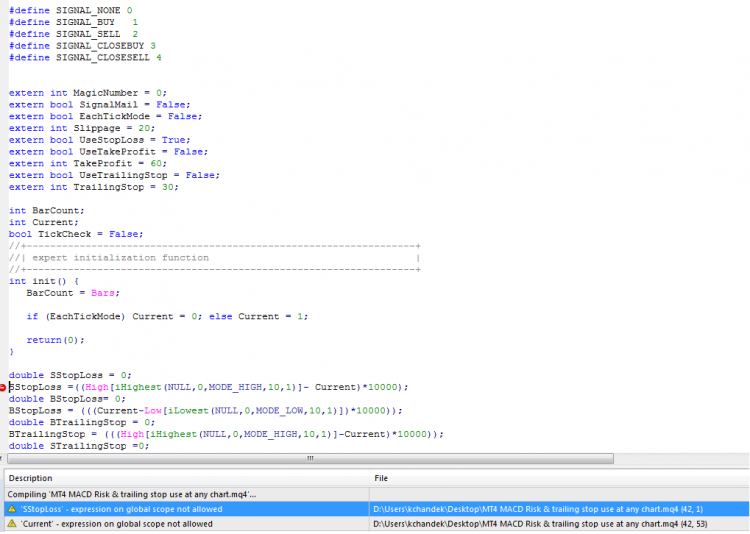# expression on global scope not allowed.1367Thanks,

Kcfve12317321

kcfve123:

I asked you to use the SRC button when posting code . . .

The code that has the problem . . . what function is it meant to be inside ? code has to be inside a function. The only thing that can be outside a function of constant declarations, variable declarations with global scope, include and library commands1367

RaptorUK:

I asked you to use the SRC button when posting code . . .

The code that has the problem . . . what function is it meant to be inside ? code has to be inside a function. The only thing that can be outside a function of constant declarations, variable declarations with global scope, include and library commands

```#define SIGNAL_NONE 0
#define SIGNAL_SELL  2
#define SIGNAL_CLOSESELL 4

extern int MagicNumber = 0;
extern bool SignalMail = False;
extern bool EachTickMode = False;
extern int Slippage = 20;
extern bool UseStopLoss = True;
extern bool UseTakeProfit = False;
extern int TakeProfit = 60;
extern bool UseTrailingStop = False;
extern int TrailingStop = 30;

int BarCount;
int Current;
bool TickCheck = False;
//+------------------------------------------------------------------+
//| expert initialization function                                   |
//+------------------------------------------------------------------+
int init() {
BarCount = Bars;

if (EachTickMode) Current = 0; else Current = 1;

return(0);
}

double SStopLoss = 0;
SStopLoss =((High[iHighest(NULL,0,MODE_HIGH,10,1)]- Current)*10000);```17321

kcfve123:

I already did . . . what is the answer to my question ?

RaptorUK:

I asked you to use the SRC button when posting code . . .

The code that has the problem . . . what function is it meant to be inside ?

What function does this code belong to ?

`SStopLoss =((High[iHighest(NULL,0,MODE_HIGH,10,1)]- Current)*10000);`1367

I want calculate stoploss based on some condition like for sell position it will look for last 10 candels highest value & check difference with current price at which trade will get open. Then it will set stop loss as high value.

This difference pips will also be used for lot size calculation depend on % of risk so below code

`SStopLoss =((High[iHighest(NULL,0,MODE_HIGH,10,1)]- Current)*10000);`

.23788

kcfve123: I want...
What you want is irrelevant. Previously asked: "What function does that line of code belong in." It is currently not in any function. Answer the question.17321

kcfve123:

I want calculate stoploss based on some condition like for sell position it will look for last 10 candels highest value & check difference with current price at which trade will get open. Then it will set stop loss as high value.

This difference pips will also be used for lot size calculation depend on % of risk so below code

.

Oh dear . . .

Do this . . .

```double SStopLoss = 0;   // move these lines up here before init()

//+------------------------------------------------------------------+
//| expert initialization function                                   |
//+------------------------------------------------------------------+
int init() {
BarCount = Bars;

if (EachTickMode) Current = 0; else Current = 1;

return(0);
}

int start()
{
SStopLoss =((High[iHighest(NULL,0,MODE_HIGH,10,1)]- Current)*10000);   // add these lines into the start() function . . .
```1367

Thanks Raptor you are too good.